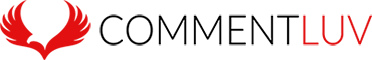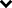# Brewsterware

## August 22, 2010

### strptime for windows

Filed under: PHP and MySQL — Joe Brewer @ 2:36 pm

I was searching for a windows implementation for the php function strptime, however the best help/advice that I could find was read the source code and write it yourself. Here is my implentation that I knocked up with date_parse_from_format so you can use it on version of php less than 5.3.0

```if (!function_exists('date_parse_from_format')) {
function date_parse_from_format(\$format, \$date) {
\$returnArray = array('hour' => 0, 'minute' => 0, 'second' => 0,
'month' => 0, 'day' => 0, 'year' => 0);

\$dateArray = array();

// array of valid date codes with keys for the return array as the values
\$validDateTimeCode = array('Y' => 'year', 'y' => 'year',
'm' => 'month', 'n' => 'month',
'd' => 'day', 'j' => 'day',
'H' => 'hour', 'G' => 'hour',
'i' => 'minute', 's' => 'second');

/* create an array of valid keys for the return array
* in the order that they appear in \$format
*/
for (\$i = 0 ; \$i <= strlen(\$format) - 1 ; \$i++) {
\$char = substr(\$format, \$i, 1);

if (array_key_exists(\$char, \$validDateTimeCode)) {
\$dateArray[\$validDateTimeCode[\$char]] = '';
}
}

// create array of reg ex things for each date part
\$regExArray = array('.' => '\.', // escape the period

// parse d first so we dont mangle the reg ex
// day
'd' => '(\d{2})',

// year
'Y' => '(\d{4})',
'y' => '(\d{2})',

// month
'm' => '(\d{2})',
'n' => '(\d{1,2})',

// day
'j' => '(\d{1,2})',

// hour
'H' => '(\d{2})',
'G' => '(\d{1,2})',

// minutes
'i' => '(\d{2})',

// seconds
's' => '(\d{2})');

// create a full reg ex string to parse the date with
\$regEx = str_replace(array_keys(\$regExArray),
array_values(\$regExArray),
\$format);

// Parse the date
preg_match("#\$regEx#", \$date, \$matches);

// some checks...
if (!is_array(\$matches) ||
\$matches != \$date ||
sizeof(\$dateArray) != (sizeof(\$matches) - 1)) {
return \$returnArray;
}

// an iterator for the \$matches array
\$i = 1;

foreach (\$dateArray AS \$key => \$value) {
\$dateArray[\$key] = \$matches[\$i++];

if (array_key_exists(\$key, \$returnArray)) {
\$returnArray[\$key] = \$dateArray[\$key];
}
}

return \$returnArray;
}
}

if (!function_exists('strptime')) {
function strptime(\$format, \$date) {
\$dateArray = array();

\$dateArray = date_parse_from_format(\$format, \$date);

if (is_array(\$dateArray)) {
return mktime(\$dateArray['hour'],
\$dateArray['minute'],
\$dateArray['second'],
\$dateArray['month'],
\$dateArray['day'],
\$dateArray['year']);
} else {
return 0;
}
}
}```

1.Genius. Saved me some effort!

Comment by jim — July 15, 2011 @ 11:18 am

2.Comment by Joe Brewer — July 15, 2011 @ 3:57 pm

3.Hello…this is great! I was writing one myself but I stumbled upon this…it fits my needs very well. But I did some changes to reflect more my needs and to resemble more the original one provided with php 5.3
I simply added error_count and warning_count into the resulting array and added error_count = 1 when regexp fails and warning_count = 1 when the date is not valid (eg. 32/01/2010 or leap year problem). So in your code you can simply write
\$d = date_parse_from_format(‘j-n-Y’, \$strd);
if(\$d[‘error_count’]>0) { ..error… }

Hope it helped as yours did. Thank you.

```if (!function_exists('date_parse_from_format')) {
function date_parse_from_format(\$format, \$date) {
\$returnArray = array('hour' => 0, 'minute' => 0, 'second' => 0,
'month' => 0, 'day' => 0, 'year' => 0,
'error_count' => 0, 'warning_count' => 0);

\$dateArray = array();

// array of valid date codes with keys for the return array as the values
\$validDateTimeCode = array('Y' => 'year', 'y' => 'year',
'm' => 'month', 'n' => 'month',
'd' => 'day', 'j' => 'day',
'H' => 'hour', 'G' => 'hour',
'i' => 'minute', 's' => 'second');

/* create an array of valid keys for the return array
* in the order that they appear in \$format
*/
for (\$i = 0 ; \$i <= strlen(\$format) - 1 ; \$i++) {
\$char = substr(\$format, \$i, 1);

if (array_key_exists(\$char, \$validDateTimeCode)) {
\$dateArray[\$validDateTimeCode[\$char]] = '';
}
}

// create array of reg ex things for each date part
\$regExArray = array('.' => '\.', // escape the period

// parse d first so we dont mangle the reg ex
// day
'd' => '(\d{2})',

// year
'Y' => '(\d{4})',
'y' => '(\d{2})',

// month
'm' => '(\d{2})',
'n' => '(\d{1,2})',

// day
'j' => '(\d{1,2})',

// hour
'H' => '(\d{2})',
'G' => '(\d{1,2})',

// minutes
'i' => '(\d{2})',

// seconds
's' => '(\d{2})');

// create a full reg ex string to parse the date with
\$regEx = str_replace(array_keys(\$regExArray),
array_values(\$regExArray),
\$format);

// Parse the date
preg_match("#\$regEx#", \$date, \$matches);

// some checks...
if (!is_array(\$matches) ||
\$matches != \$date ||
sizeof(\$dateArray) != (sizeof(\$matches) - 1)) {
\$returnArray['error_count'] = 1;
return \$returnArray;
}

// an iterator for the \$matches array
\$i = 1;

foreach (\$dateArray AS \$key => \$value) {
\$dateArray[\$key] = \$matches[\$i++];

if (array_key_exists(\$key, \$returnArray)) {
\$returnArray[\$key] = \$dateArray[\$key];
}
}

if(!checkdate(\$returnArray['month'], \$returnArray['day'], \$returnArray['year'])) {
\$returnArray['warning_count'] = 1;
}

return \$returnArray;
}
}
```

Comment by Crick — March 23, 2012 @ 4:25 pm

4.Awesome – thanks for sharing.

Comment by Joe Brewer — March 23, 2012 @ 5:27 pm

5.Thanks for sharing this great function!

Comment by Mike — October 10, 2012 @ 8:11 pm

6.BIG THANK’S!

Comment by Serguei — December 12, 2012 @ 6:05 am